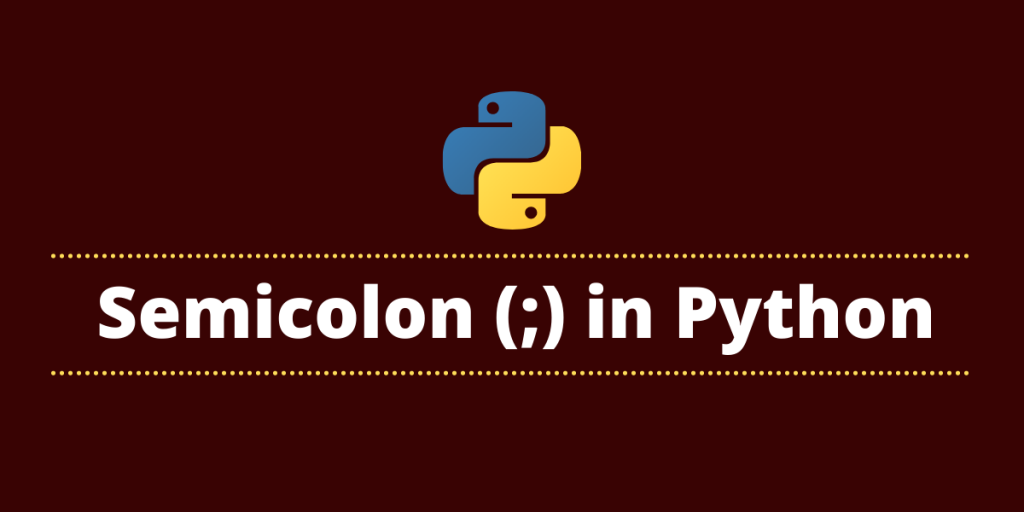# Semicolon in PythonThe common meaning of a semicolon(;) in various programming languages is to end or discontinue the current statement. In programming languages such as C, C++, and Java, it is necessary to use a semicolon to end a line of code. However, this is not the case with Python.

A semicolon in Python signifies separation rather than termination. It allows you to write multiple statements on a single line.

There are many use cases of semicolons than just mentioned above, in this tutorial we will see different uses of semicolons in Python and understand it better with examples.

## Why are semicolons allowed in Python?

Python does not require semi-colons to terminate statements. Semicolons can be used to delimit statements if you wish to put multiple statements on the same line.

semicolon in Python denotes separation, rather than termination. It allows you to write multiple statements on the same line. This syntax also makes it legal to put a semicolon at the end of a single statement. So, it’s actually two statements and the second one is empty.

## How to print a semicolon in Python?

Many people consider the semicolon to be different from other alphabets. Let’s see what happens when we try to print a semicolon as a regular string in Python

```>>> print(";")
```

Output:

```;
```

It treats the semicolon no differently and prints it out.

## Split Statements with Semicolons

Sometimes there is a need to write multiple statements in a line to make the code single liners or for some other reason, in this type of case semicolons are very useful. A semicolon can be used to separate statements in Python.

Below is the syntax for using a semicolon to separate two statements, but these statements can be more than two.

Syntax:

```statement1; statement2
```

Example:

In this example, we will try to put more than 2 statements in a single line with the use of the semicolon.

Here are the three statements in Python without semicolons:

```>>> print('Hi')
>>> print('Hello')
>>> print('Hola!')
```

Now let’s use the same three statements with semicolons

```print('Hi'); print('Hello'); print('Hola!')
```

Output:

```Hi
Hello
Hola!
```

As you can see, Python executes the three statements individually after we split them with semicolons. Without the use of that, the interpreter would give us an error.

## Using Semicolons with Loops in Python

In loops like the For loop, a semicolon can be used if the whole statement starts with a loop. You use a semicolon to form a coherent statement like the body of the loop.

Example:

In this example, we’ll try to loop through two statements separated by a semicolon and see if the loop prints both or just the first one.

```for i in range (4): print ('Hi') ; print('Hello')
```

Here we have used the range() method to specify a loop to execute statements four times.

Output:

```Hi
Hello
Hi
Hello
Hi
Hello
Hi
Hello
```

In the above output, we can see that it successfully prints both statements the specified number of times, which means it can loop through all the statements separated by semicolons.

Python will throw an error if you use a semicolon to separate a normal expression from a block statement i.e loop.

```print('Hi') ; for i in range (4): print ('Hello')
```

Output:

```Invalid Syntax
```

## Conclusion

In this tutorial, we have learned various use cases of a semicolon in Python. Let’s summarize them with two pointers:

• A semicolon in Python is mostly used to separate multiple statements written on a single line.
• A semicolon is used to write a minor statement and reserve a bit of space like name = Marie; age = 23; print(name, age)

The use of semicolons is very “non-pythonic” and it is recommended not to be used unless you desperately need it.

## Reference

https://stackoverflow.com/questions/8236380/why-is-semicolon-allowed-in-this-python-snippet